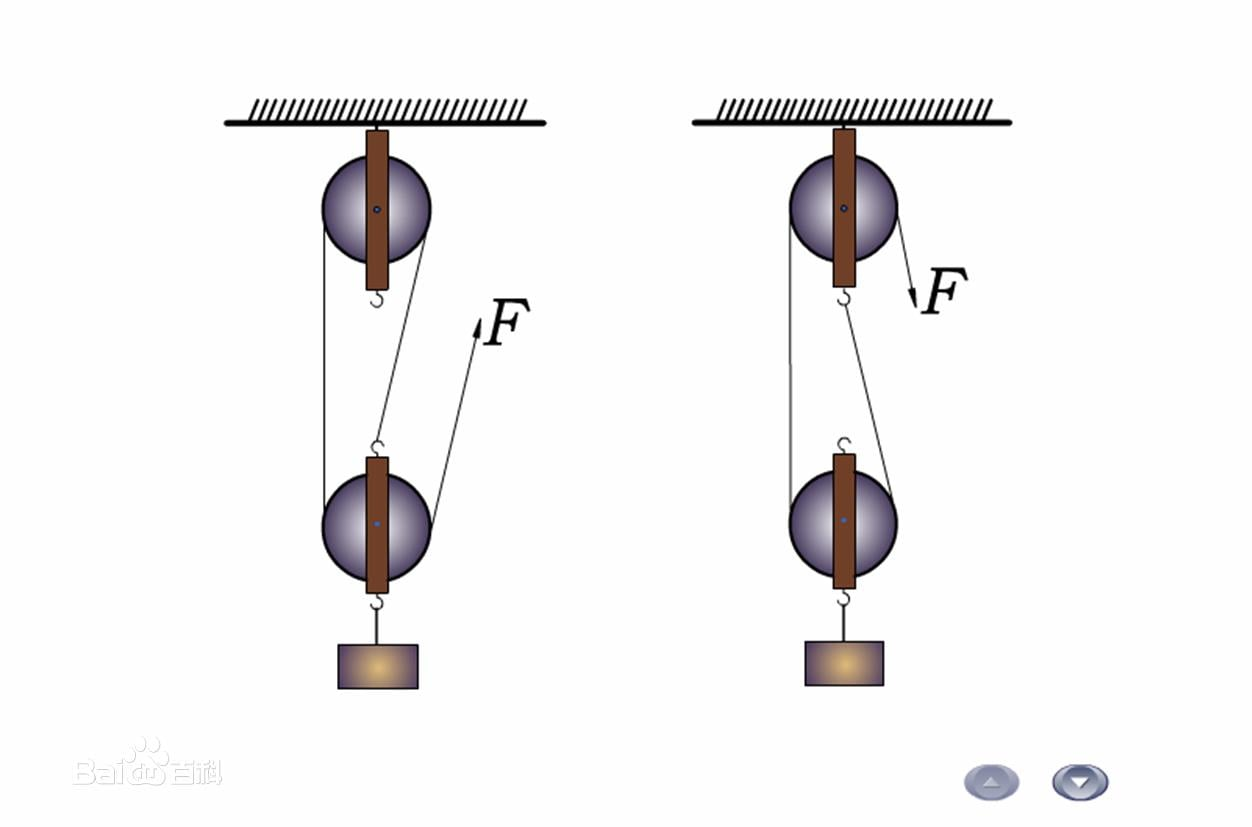## General Mechanical

•hustfocus
Subscriber
excuse me,my english is poor.what elements are chosen for rope and pulley ?rope and pulley how to define constraints?
•peteroznewman
Subscriber

Please explain the purpose of your analysis.

What do you want to know about the rope and pulley?

•hustfocus
Subscriber
Analyze the motion and deformation of a fixed pulley block. The structure consists of a fixed pulley and a rope around it, with a weight at one end and a loading force at the other end to analyze the motion and deformation of the rope and weight.The sliding constraints between the rope and the pulley don't know how to define the effect of the rope on the pulley.Thanks.
•peteroznewman
Subscriber

The best practice is to build the simplest model that will give you some useful information, even though it may not give you all the information you ultimately want to know. The benefit of the simple model is you will learn how to get it working and see the main effect without spending too much time on details that only have a secondary effect on the results. Here is a list of questions you should have the answers to build a useful model.

• Do you have solid body geometry for the pulley from some CAD system like SolidWorks or SpaceClaim?

• Do you need to analyze a specific pulley geometry or are you free to design your own pulley?

• What is the diameter of the pulley at the centerline of the rope?

• What is the diameter of the hole in the pulley that rests on the pin through the center?

• What is the diameter of the rope?

• What is the material of the pulley?

• What is the yield strength of the pulley material?

• What is the material of the rope?

• What is the construction of the rope?

• What is the rated strength of the rope?

• What is the material of the pin in the center of the pulley?

• What is the diameter of the pin?

• What is the coefficient of friction between the pin and the pulley?

• What is the coefficient of friction between the rope and the pulley?

• What is the weight supported by the rope?

The simplest model would have just a pulley, no rope, no pin. Use symmetry to slice the pulley in half separating the two side that have the rope exiting the pulley. Apply a compression only support to the face of the half hole at the center, and apply a bearing load to the face of the half pulley equal to the weight. That will show you the main effect of the stress in the pulley.  You can even use symmetry to slice the pulley to half the thickness if it is symmetric through the thickness. If you do that, then you only apply half the weight.

There are second order effects due to the friction of the rope on the pulley, but get that simple model working first.

Regards,
Peter

•hustfocus
Subscriber

Thanks.But I want to know is how to define the relationship between the pulley and ropes(such as contact), so that I can simulate the deformation and axial force and the stress when the movement of the rope on the pulley, As shown in the figure below•peteroznewman
Subscriber

You are showing two pulley systems, the tension in the rope is shown to be equal to F, so one system is supporting a heavier weight than the other.

A pulley is made up of a sheave, the round part that the rope touches, two flat parts, the shaft between them, and the hooks on either end. Let's use the name frame for the assembly of parts excluding the sheave.

For both systems, there is approximately a force of 2F downward (or upward) on the sheave. Each system has an additional force on the frame where the rope terminates.

The stress on the sheave doesn't change much whether is is static or moving, other than the sheave would be at different angles as it the system moves, but if the sheave is rotationally symmetric (has no spokes, is a solid shape) that makes no difference.

"how to define the relationship between the pulley and ropes"

I suggested starting with a simple model, which applies a force of 2F to the sheave, why don't you start there?  That simple model will "simulate the deformation and axial force and the stress" on the sheave.

If you want to add in the rope, then use frictional contact between the rope and sheave, but it will make the model a lot more difficult to solve.

Regards,
Peer

•hustfocus
Subscriber

Thanks very much.